Thank you for visiting nature.com. You are using a browser version with limited support for CSS. To obtain the best experience, we recommend you use a more up to date browser (or turn off compatibility mode in Internet Explorer). In the meantime, to ensure continued support, we are displaying the site without styles and JavaScript.

Abstract

In the current era of noisy intermediate-scale quantum computers, noisy qubits can result in biased results for early quantum algorithm applications. This is a significant challenge for interpreting results from quantum computer simulations for quantum chemistry, nuclear physics, high energy physics (HEP), and other emerging scientific applications. An important class of qubit errors are readout errors. The most basic method to correct readout errors is matrix inversion, using a response matrix built from simple operations to probe the rate of transitions from known initial quantum states to readout outcomes. One challenge with inverting matrices with large off-diagonal components is that the results are sensitive to statistical fluctuations. This challenge is familiar to HEP, where prior-independent regularized matrix inversion techniques (“unfolding”) have been developed for years to correct for acceptance and detector effects, when performing differential cross section measurements. We study one such method, known as iterative Bayesian unfolding, as a potential tool for correcting readout errors from universal gate-based quantum computers. This method is shown to avoid pathologies from commonly used matrix inversion and least squares methods.

Introduction

While quantum algorithms are promising techniques for a variety of scientific and industrial applications, current challenges limit their immediate applicability. One significant limitation is the rate of errors and decoherence in noisy intermediate-scale quantum (NISQ) computers1. Mitigating errors is hard in general because quantum bits (“qubits”) cannot be copied2,3,4. An important family of errors are readout errors. They typically arise from two sources: (1) measurement times are significant in comparison to decoherence times, and thus a qubit in the $$\left|1\right\rangle$$ state can decay to the $$\left|0\right\rangle$$ state during a measurement, and (2) probability distributions of measured physical quantities that correspond to the $$\left|0\right\rangle$$ and $$\left|1\right\rangle$$ states have overlapping support, and there is a small probability of measuring the opposite value. The goal of this paper is to investigate methods for correcting these readout errors. This is complementary to efforts for gate error corrections. One strategy for mitigating such errors is to build in error correcting components into quantum circuits. Quantum error correction5,6,7,8,9 is a significant challenge because qubits cannot be cloned2,3,4. This generates a significant overhead in the additional number of qubits and gates required to detect or correct errors. Partial error detection/correction has been demonstrated for simple quantum circuits10,11,12,13,14,15,16,17,18,19, but complete error correction is infeasible for current qubit counts and moderately deep circuits. As a result, many studies with NISQ devices use the alternative zero noise extrapolation strategy, whereby circuit noise is systematically increased and then extrapolated to zero20,21,22,23,24,25. Ultimately, both gate and readout errors must be corrected for a complete measurement and a combination of strategies may be required.

Correcting measured histograms for the effects of a detector has a rich history in image processing, astronomy, high energy physics (HEP), and beyond. In the latter, the histograms represent binned differential cross sections and the correction is called unfolding. Many unfolding algorithms have been proposed and are currently in use by experimental high energy physicists (see, e.g., refs. 26,27,28 for reviews). One of the goals of this paper is to introduce these methods and connect them with current algorithms used by the quantum information science (QIS) community.

Quantum readout error correction can be represented as histogram unfolding, where each bin corresponds to one of the possible $${2}^{{n}_{\text{qubit}}}$$ configurations, where nqubit is the number of qubits (Fig. 1). Correcting readout noise is a classical problem (though there has been a proposal to do it with quantum annealing29), but relies on calibrations or simulations from quantum hardware. Even though discussions of readout errors appear in many papers (see, e.g., refs. 24,30,31,32), we are not aware of any dedicated study comparing unfolding methods for QIS applications. Furthermore, current QIS methods have pathologies that can be avoided with techniques from HEP. In particular, the most popular quantum simulators pyQuil33 (by Rigetti), Cirq34,35 (by Google), and XACC36,37,38 implement a version of matrix inversion, and the other popular simulator Qiskit by IBM39,40 uses a least squares method (see also refs. 41,42) that is the same as the matrix inversion solution when the the latter is nonnegative. Challenges with methods based on matrix inversion will be discussed in more detail below.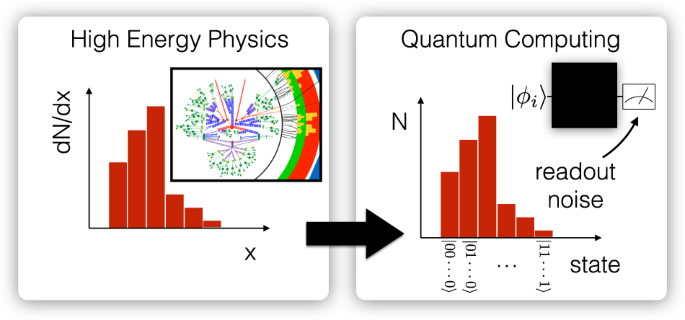Fig. 1: A schematic diagram illustrating the connection between binned differential cross section measurements in high energy physics (left) and interpreting the output of repeated measurements from quantum computers (right).

Results

For the results presented here, we simulate a quantum computer using qiskit-terra 0.9.0, qiskit-aer 0.2.3, and qiskit-ignis 0.1.139. Note that multi-qubit readout errors are not supported in all versions of qiskit-aer and qiskit-ignis. This work uses a custom measure function to implement the response matrices. We choose a Gaussian distribution as the true distribution, as this is ubiquitous in quantum mechanics as the ground state of the harmonic oscillator. An alternative distribution that is mostly zero with a small number of spikes is presented in Supplementary Fig. 5. This system has been recently studied in the context quantum field theory as a benchmark 0 + 1-dimensional noninteracting scalar field theory43,44,45,46,47,48,49,50. In practice, all of the qubits of a system would be entangled to achieve the harmonic oscillator wave function. However, this is unnecessary for studying readout errors, which act at the ensemble level. The Gaussian is mapped to qubits using the following map:

$$t(b)\propto \exp \left[-\frac{1}{2\sigma }{\left(b-{2}^{{n}_{\text{qubit}}-1}\right)}^{2}\right],$$
(1)

where b is the binary representation of a computational basis state, i.e., $$\left|00000\right\rangle\, \mapsto \,0$$ and $$\left|00011\right\rangle\, \mapsto \,3$$. For the results shown below, σ = 3.5.

As a first test, a pathological response matrix (see the “Methods” section) is used to illustrate a failure mode of the matrix inversion and ignis approaches. Figure 2 compares $${\hat{t}}_{\text{matrix}}$$, $${\hat{t}}_{\text{ignis}}$$, and $${\hat{t}}_{\text{IBU}}$$ for a four-qubit Gaussian distribution. As the matrix inversion result is already nonnegative, the $${\hat{t}}_{\text{ignis}}$$ result is nearly identical to $${\hat{t}}_{\text{matrix}}$$. Both of these approaches s how large oscillations. In contrast, the iterative Bayesian unfolding (IBU) method with ten iterations is nearly the same as the truth distribution. This result is largely insensitive to the choice of iteration number, though it approaches the matrix inversion result for more than about a thousand iterations. This is because the IBU method converges to a maximum likelihood estimate51, which in this case aligns with the matrix inversion result. If the matrix inversion would have negative entries, then it would differ from the asymptotic limit of the IBU method, which is always nonnegative.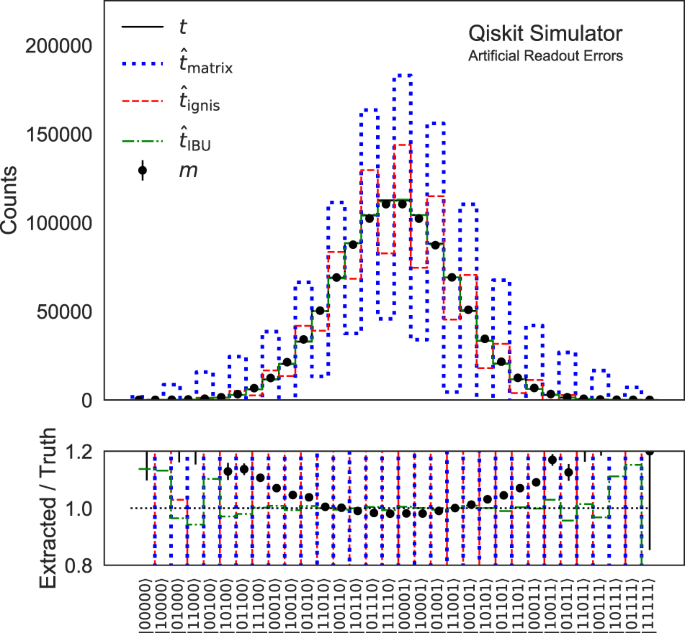Fig. 2: The measurement of a Gaussian distribution (ground state of harmonic oscillator) using the pathological response matrix.

While Fig. 2 is illustrative for exposing a potential failure mode of matrix inversion and the ignis method, it is also useful to consider a realistic response matrix. Figure 3 uses the same Gaussian example as above, but for an IBM Q Johannesburg response matrix (see the “Methods section). Even though the migrations are large, the pattern is such that all three methods qualitatively reproduce the truth distribution. The dip in the middle of the distribution is due to the mapping between the Gaussian and qubit states: all of the state to the left of the center have a zero as the last qubit, while the ones on the right have a one as the last qubit. The asymmetry of 0 → 1 and 1 → 0 induces the asymmetry in the measured spectrum. For example, it is much more likely to migrate from any state to $$\left|00000\right\rangle$$ than to $$\left|11111\right\rangle$$.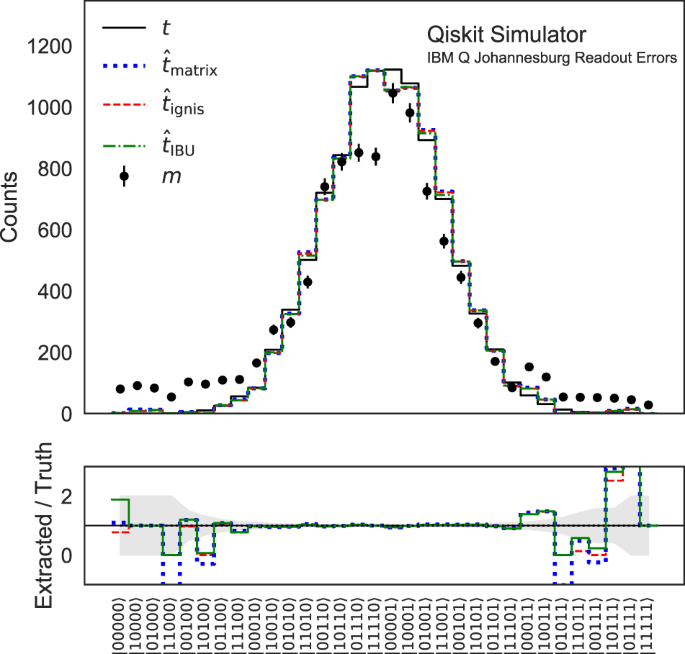Fig. 3: The measurement of a Gaussian distribution using the response matrix from the IBM Q Johannesburg machine.

The three unfolding approaches are quantitatively compared in Fig. 4. The same setup as Fig. 2 is used, repeated over 1000 pseudo-experiments. For each of the 1000 pseudo-experiments, a discretized Gaussian state is prepared (same as Fig. 2) with no gate errors and it is measured 104 times. The bin counts without readout errors are the “true” values. These values include the irreducible Poisson noise that the unfolding methods are not expected to correct. The measured distribution with readout errors is unfolded using the three method, and the resulting counts are the “predicted” values. Averaging over many pseudo-experiments, the spread in the predictions is a couple of percent smaller for $${\hat{t}}_{\text{IBU}}$$ compared to $${\hat{t}}_{\text{ignis}}$$, and both of these are ~10% more precise than $${\hat{t}}_{\text{matrix}}$$. The slight bias of $${\hat{t}}_{\text{ignis}}$$ and $${\hat{t}}_{\text{IBU}}$$ to the right results from the fact that they are nonnegative. Similarly, the sharp peak at zero results from $${\hat{t}}_{\text{ignis}}$$ and $${\hat{t}}_{\text{IBU}}$$ values that are nearly zero when ti ~ 0. In contrast, zero is not special for matrix inversion so there is no particular feature in the center of its distribution.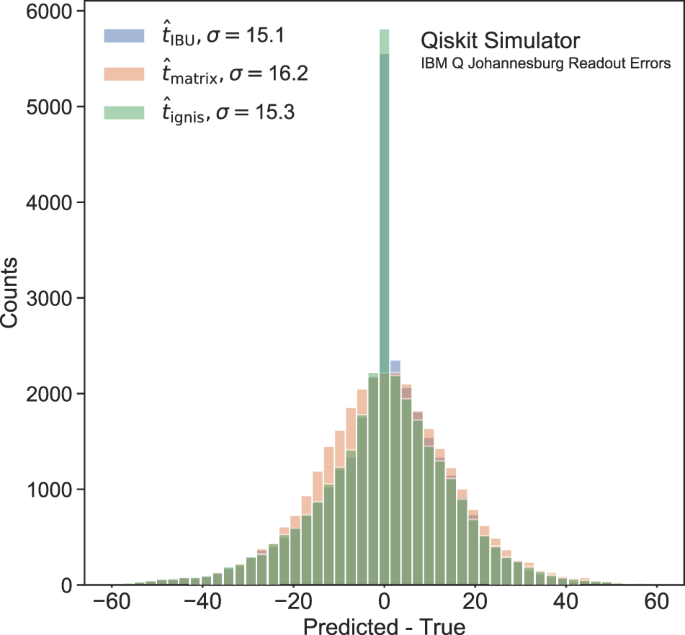Fig. 4: The distribution of the difference between true and predicted counts from a Gaussian distribution using the response matrix from the IBM Q Johannesburg machine.

Regularization and uncertainties

One feature of any regularization method is the choice of regularization parameters. For the IBU method, these parameters are the prior and number of iterations. Figure 5 shows the average bias from statistical and systematic sources in the measurement from the Gaussian example shown in Fig. 2, as a function of the number of iterations. With a growing number of iterations, $${\hat{t}}_{\text{IBU}}$$ approaches the oscillatory $${\hat{t}}_{\text{ignis}}$$. The optimal number of iterations from the point or view of the bias is three. However, the number of iterations cannot be chosen based on the actual bias because the true answer is not known a priori. In HEP, the number of iterations is often chosen before unblinding the data by minimizing the total expected uncertainty. In general, there are three sources of uncertainty: statistical uncertainty on m, statistical and systematic uncertainties on R, and non-closure uncertainties from the unfolding method. Formulae for the statistical uncertainty on m are presented in ref. 52 and can also be estimated by bootstrapping53 the measured counts. Similarly, the statistical uncertainty on R can be estimated by bootstrapping and then repeating the unfolding for each calibration pseudo-dataset. The sources of statistical uncertainty are shown as dot-dashed and dashed lines in Fig. 6. Adding more iterations enhances statistical fluctuations and so these sources of uncertainty increase monotonically, with the number of iterations.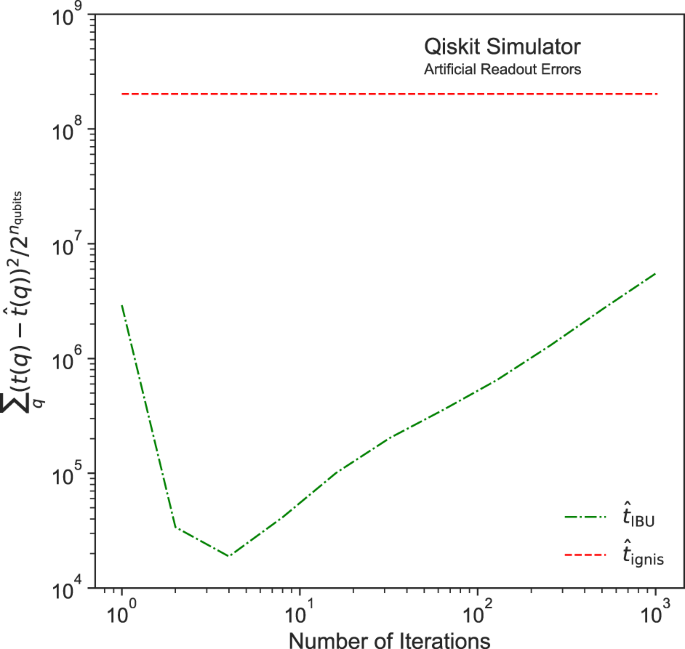Fig. 5: The difference between $${\hat{t}}_{\text{IBU}}$$ and t as a function of the number of iterations for the example presented in Fig. 2.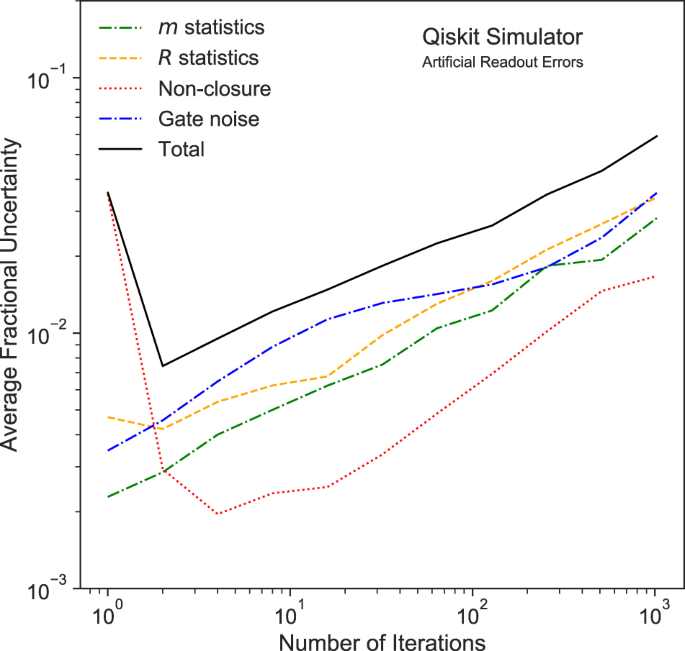Fig. 6: Sources of uncertainty for $${\hat{t}}_{\text{IBU}}$$ as a function of the number of iterations for the example presented in Fig. 2.

The systematic uncertainty on R and the method non-closure uncertainty are not unique and require careful consideration. In HEP applications, R is usually determined from simulation, so the systematic uncertainties are simulation variations that try to capture potential sources of mis-modeling. These simulation variations are often estimated from auxiliary measurements with data. There are additional uncertainties related to the modeling of background processes, as well as related to events that fall into or out of the measurement volume. These are not relevant for QIS. In the QIS context, R is determined directly from the data, so the only uncertainty is on the impurity of the calibration circuits. In particular, the calibration circuits are constructed from a series of single-qubit X gates. Due to gate imperfections and thermal noise, there is a chance that the application of an X gate will have a different effect on the state than intended. In principle, one can try to correct for such potential sources of bias by an extrapolation method. In such methods, the noise is increased in a controlled fashion and then extrapolated to zero noise20,21,22,23,24. This method may have a residual bias and the uncertainty on the method would then become the systematic uncertainty on R. A likely conservative alternative to this approach is to modify R by adding in gate noise, and taking the difference between the nominal result and one with additional gate noise, simulated using the thermal_relaxation_error functionality of qiskit. This is the choice made in Fig. 6, where the gate noise is shown as a dot-dashed line. In this particular example, the systematic uncertainty on R increases monotonically with the number of iterations, just like the sources of statistical uncertainty.

The non-closure uncertainty is used to estimate the potential bias from the unfolding method. One possibility is to compare multiple unfolding methods and take the spread in predictions as an uncertainty. Another method advocated in ref. 54 and widely used in HEP is to perform a data-driven reweighting. The idea is to reweight the t0 so that when folded with R, the induced m0 is close to the measurement m. Then, this reweighted m0 is unfolded with the nominal response matrix and compared with the reweighted t0. The difference between these two is an estimate of the non-closure uncertainty. The reweighting function is not unique, but should be chosen so that the reweighted t0 is a reasonable prior for the data. For Fig. 6, the reweighting is performed using the nominal unfolded result itself. In practice, this can be performed in a way that is blinded from the actual values of $${\hat{t}}_{\text{IBU}}$$ so that the experimenter is not biased, when choosing the number of iterations.

Altogether, the sources of uncertainty presented in Fig. 6 show that the optimal choice for the number of iterations is 2. In fact, the difference in the uncertainty between two and three iterations is <1% and so consistent with the results from Fig. 5. Similar plots for the measurement in Fig. 3 can be found in Supplementary Figs. 3 and 4.

Discussion

This work has introduced a suite of readout error correction algorithms developed in HEP for binned differential cross section measurements. These unfolding techniques are well-suited for quantum computer readout errors, which are naturally binned and without acceptance effects (counts are not lost or gained during readout). In particular, the iterative Bayesian method has been described in detail, and shown to be robust to a failure mode of the matrix inversion and ignis techniques. When readout errors are sufficiently small, all the methods perform well, with a preference for the ignis and Bayesian methods that produce nonnegative results. The ignis method is a special case of the TUnfold algorithm, where the latter uses the covariance matrix to improve precision and incorporates regularization to be robust to the failure modes of matrix inversion. It may be desirable to augment the ignis method with these features or provide the iterative method as an alternative approach. In either case, Fig. 3 showed that even with a realistic response matrix, readout error corrections can be significant and must be accounted for in any measurement on near-term hardware.

An important challenge facing any readout error correction method is the exponential resources required to construct the full R matrix (see the “Methods” section). While R must be constructed only once per hardware setup and operating condition, it could become prohibitive when the number of qubits is large. On hardware with few connections between qubits, per-qubit transition probabilities may be sufficient for accurate results. When that is not the case, one may be able to achieve the desired precision with polynomially many measurements. These ideas are left to future studies.

Another challenge is optimizing the unfolding regularization. The numerical examples presented in the “Results” section considered various sources of uncertainty, and studied how they depend on the number of iterations in the IBU method. The full measurement is performed for all $${2}^{{n}_{\text{qubit}}}$$ states and the studies in the “Results” section collapsed the uncertainty across all bins into a single number by averaging across bins. This way of choosing a regularization parameter is common in HEP, but is not a unique approach. Ultimately, a single number is required for optimization, but it may be that other metrics are more important for specific applications, such as the uncertainty for a particular expectation value, or the maximum or most probable uncertainty across states. Such requirements are application specific, but should be carefully considered prior to the measurement.

With active research and development across a variety of application domains, there are many promising applications of quantum algorithms in both science and industry on NISQ hardware. Readout errors are an important source of noise that can be corrected to improve measurement fidelity. HEP experimentalists have been studying readout error correction techniques for many years under the term unfolding. These tools are now available to the QIS community and will render the correction procedure more robust to resolution effects, in order to enable near-term breakthroughs.

Methods

The unfolding challenge

Let t be a vector that represents the true bin counts before the distortions from detector effects (HEP) or readout noise (QIS). The corresponding measured bin counts are denoted by m. These vectors are related by a response matrix R as m = Rt, where Rij = Pr(m = it = j). In HEP, the matrix R is usually estimated from detailed detector simulations, while in QIS, R is constructed from measurements of computational basis states. The response matrix construction is discussed in more detail below.

The most naive unfolding procedure would be to simply invert the matrix R: $${\hat{t}}_{\text{matrix}}={R}^{-1}m$$. However, simple matrix inversion has many known issues. Two main problems are that $${\hat{t}}_{\text{matrix}}$$ can have unphysical entries, and that statistical uncertainties in R can be amplified and can result in oscillatory behavior. For example, consider the case

$$R=\left(\begin{array}{ll}1-\epsilon &\epsilon \\ \epsilon &1-\epsilon \end{array}\right),$$
(2)

where 0 < ϵ < 1/2. Then, $$\,\text{Var}\,({\hat{t}}_{\text{matrix}})\propto 1/\det (R)=1/(1-2\epsilon)\to \infty$$ as ϵ → 1/2. As a generalization of this example to more bins (from ref. 55), consider a response matrix with a symmetric probability of migrating one bin up or down,

$$R=\left(\begin{array}{llll}1-\epsilon &\epsilon &0&\cdots \\ \epsilon &1-2\epsilon &\epsilon &\cdots \\ 0&\epsilon &1-2\epsilon &\cdots \\ \vdots &\vdots &\vdots &\ddots \end{array}\right).$$
(3)

Unfolding with the above response matrix and ϵ = 25% is presented in Fig. 7. The true bin counts have the Gaussian distribution with a mean of zero and a standard deviation of 3. The values are discretized with 21 uniform unit width bins spanning the interval [−10, 10]. The leftmost bin correspond to the first index in m and t. The indices are monotonically incremented with increasing bin number. The first and last bins include underflow and overflow values, respectively. Due to the symmetry of the migrations, the true and measured distributions are nearly the same. For this reason, the measured spectrum happens to align with the true distribution; the optimal unfolding procedure should be the identity. The significant off-diagonal components result in an oscillatory behavior and the statistical uncertainties are also amplified by the limited size of the simulation dataset used to derive the response matrix.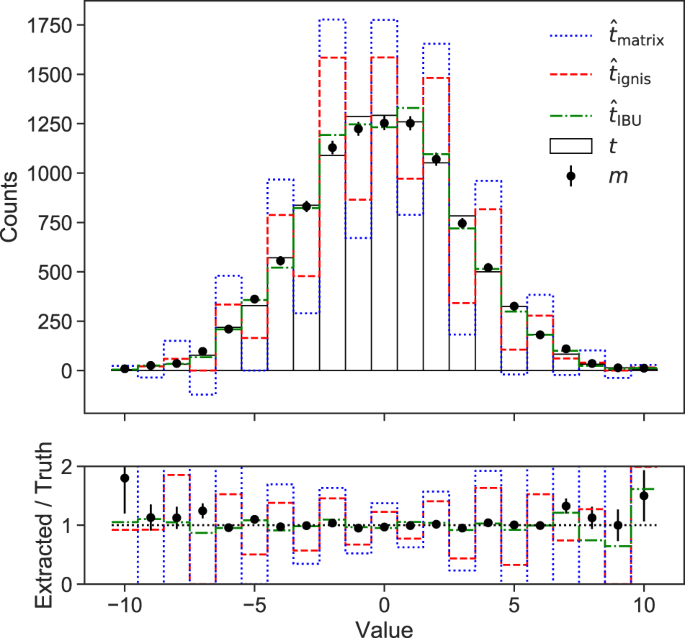Fig. 7: A comparison of unfolding techniques for a Gaussian example and the R matrix from Eq. (3).

Even though it is simple, matrix inversion is an important benchmark for comparing with the methods described below because it is widely used in quantum computing, as introduced at the end of the “Introduction” section. Many of these implementations are even simpler than full matrix inversion by assuming that errors are uncorrelated across qubits. In some cases, additional mitigation strategies are used to make the matrix inversion corrections smaller. This is demonstrated in ref. 35 (see also ref. 56), where a symmetrization step is first applied with X gates prior to matrix inversion. A minimal modification to matrix inversion is to ensure that the counts are nonnegative. One such approach is to use a least squares method40,41,42 that will be described in more detail in the next section.

Unfolding methods

The fact that matrix inversion can result in unphysical outcomes ($${\hat{t}}_{i}\,<\,0$$ or $${\hat{t}}_{i}\,>\,| | t| {| }_{1}$$, where x1 = ∑ixi is the L1 norm) is often unacceptable. One solution is to find a vector that is as close to $$\hat{t}$$ as possible, but with physical entries. This is the method implemented in qiskit-ignis39,40, a widely used quantum computation software package. The ignis method solves the optimization problem

$${\hat t}_{\rm{ignis}}={\mathop {{\rm{arg}} \, \min} \limits_{t^{\prime}{:}\Vert t^{\prime} \Vert_{1}=\Vert m \Vert_{1},\, t^{\prime} > 0}}\Vert m-Rt^{\prime}\Vert^{2}.$$
(4)

The same method was also recently studied by other authors as well, see, e.g., refs. 41,42. Note that the norm invariance is also satisfied for simple matrix inversion: $$| | {\hat{t}}_{\text{matrix}}| {| }_{1}=| | m| {| }_{1}$$ by construction because Rx1 = x1 for all x so in particular for y = R−1m, Ry1 = y1 implies that m1 = R−1m1. This means that $${\hat{t}}_{\text{ignis}}={\hat{t}}_{\text{matrix}}$$ whenever the latter is nonnegative, and so $${\hat{t}}_{\text{ignis}}$$ inherits some of the pathologies of $${\hat{t}}_{\text{matrix}}$$.

Three commonly used unfolding methods in HEP are IBU52 (also known as Richardson–Lucy deconvolution57,58), singular value decomposition (SVD) unfolding59, and TUnfold60. There are other less widely used methods, such as fully Bayesian unfolding61 and others62,63,64,65,66. Even though IBU calls for the repeated application of Bayes’ theorem, this is not a Bayesian method as there is no prior/posterior over possible distributions, only a prior for initialization. TUnfold is similar to Eq. (4), but imposes further regularization requirements in order to avoid pathologies from matrix inversion. The SVD approach applies some regularization directly on R before applying matrix inversion. The focus of this paper will be on the widely used IBU method, which avoids fitting and matrix inversion altogether with an iterative approach. Reference 52 also suggested that one could combine the iterative method with smoothing from a fit, in order to suppress amplified statistical fluctuations.

Given a prior truth spectrum $${t}_{i}^{0}=\Pr (\,\text{truth is}\,i\text{}\,)$$, the IBU technique proceeds according to the equation

$$\begin{array}{ll}{t}_{i}^{n+1}&=\mathop{\sum }\limits_{j}\Pr (\,{\text{truth}}\, {\text{is}}\,{i}| {\text{measure}}\,j)\times {m}_{j}\\ &=\mathop{\sum }\limits_{j}\frac{{R}_{ji}{t}_{i}^{n}}{{\sum }_{k}{R}_{jk}{t}_{k}^{n}}\times {m}_{j},\end{array}$$
(5)

where n is the iteration number and one iterates a total of N times. The advantage of Eq. (5) over simple matrix inversion is that the result is a probability (nonnegative and unit measure) when m ≥ 0. In HEP applications, m can have negative entries resulting from background subtraction. In this case, the unfolded result can also have negative entries. The parameters $${t}_{i}^{0}$$ and N must be specified ahead of time. A common choice for $${t}_{i}^{0}$$ is the uniform distribution. The number of iterations needed to converge depends on the desired precision, how close $${t}_{i}^{0}$$ is to the final distribution, and the importance of off-diagonal components in R. In practice, it may be desirable to choose a relatively small N prior to convergence to regularize the result. Typically, $$\lesssim {\mathcal{O}}(10)$$ iterations are needed.

In addition to the $${\hat{t}}_{\text{matrix}}$$ and $${\hat{t}}_{\text{ignis}}$$ approaches discussed in the previous section, Fig. 7 shows the IBU result with N = 10. Unlike the $${\hat{t}}_{\text{matrix}}$$ and $${\hat{t}}_{\text{ignis}}$$ results, the $${\hat{t}}_{\text{IBU}}$$ does not suffer from rapid oscillations and like $${\hat{t}}_{\text{ignis}}$$, is nonnegative. Analogous results for quantum computer simulations are presented in the “Results” section.

Constructing the response matrix

In practice, the R matrix is not known exactly, and must be measured for each quantum computer and set of operating conditions. One way to measure R is to construct a set of $${2}^{{n}_{\text{qubit}}}$$ calibration circuits as shown in Fig. 8. Simple X gates are used to prepare all of the possible qubit configurations and then they are immediately measured.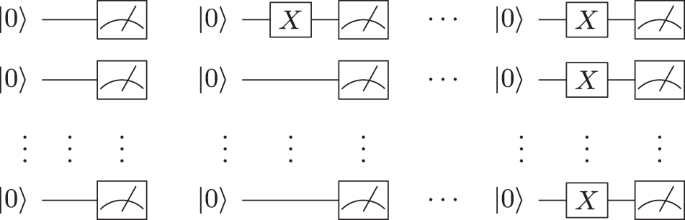Fig. 8: The set of $$2^{n_{\rm{qubit}}}$$ calibration circuits.

Figure 9 shows the R matrix from five qubits of the IBM Q Johannesburg machine. As expected, there is a significant diagonal component that corresponds to cases, when the measured and true states are the same. However, there are significant off-diagonal components, which are larger toward the right when more configurations start in the one state. The diagonal stripes with transition probabilities of ~5–7% are the result of the same qubit flipping from 0 ↔ 1. This matrix is hardware dependent and its elements can change over time due to calibration drift. Machines with higher connectivity have been observed to have more readout noise.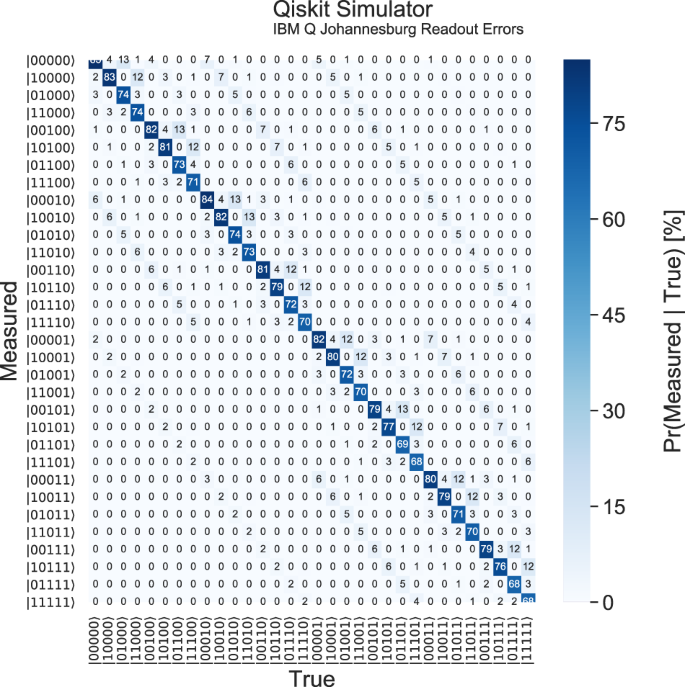Fig. 9: An example R matrix from five qubits of the IBM Q Johannesburg machine using 8192 shots for each of the 25 possible states.

Figure 10 explores the universality of qubit migrations across R. If every qubit was identical, and there were no effects from the orientation and connectivity of the computer, then one may expect that R can actually be described by just two numbers p0→1 and p1→0, the probability for a zero to be measured as a one and vice versa. These two numbers are extracted from R by performing the fit in Eq. (6):

$${\mathop {\min }\limits_{\mathop {{p_{0 \to 1}}}\limits_{{p_{1 \to 0}}}}}{\sum\limits_{ij}}{{{\left| {{R_{ij}} - p_{0 \to 1}^\alpha {{(1 - {p_{0 \to 1}})}^{{\alpha ^{\prime}}}}p_{1 \to 0}^\beta {{(1 -{p_{1 \to 0}})}^{{\beta ^{\prime}}}}} \right|}^2},}$$
(6)

where α is the number of qubits corresponding to the response matrix entry Rij that flipped from 0 to 1, $$\alpha ^{\prime}$$ is the number that remained as a 0 and $$\beta ,\beta ^{\prime}$$ are the corresponding entries for a one state; $$\alpha +\alpha ^{\prime} +\beta +\beta ^{\prime} ={n}_{\text{qubit}}$$. For example, if the Rij entry corresponds to the state $$\left|01101\right\rangle$$ migrating to $$\left|01010\right\rangle$$, then α = 1, $$\alpha ^{\prime} =1$$, β = 2, and $$\beta ^{\prime} =1$$.

A global fit to these parameters for the Johannesburg machine results in p0→1 ≈ 3.2% and p1→0 ≈ 7.5%. In reality, the qubits are not identical and so one may expect that p0→1 and p1→0 depend on the qubit. A fit to nqubit values for p0→1 and p1→0 (Eq. (6), but fitting ten parameters instead of two) are shown as filled triangles in Fig. 10. While these values cluster around the universal values (dotted lines), the spread is a relative 50% for p0→1 and 60% for p1→0. Furthermore, the transition probabilities can depend on the values of neighboring qubits. The open markers in Fig. 10 show the transition probabilities for each qubit with the other nqubit − 1 qubits held in a fixed state. In other words, the $${2}^{{n}_{\text{qubit}}-1}$$ open triangles for each qubit show

$$\Pr (|{q}_{0},\ldots ,{q}_{i},\ldots ,{q}_{{n}_{\text{qubit}}}\rangle \to |{q}_{0},\ldots ,q^{\prime} ,\ldots ,{q}_{{n}_{\text{qubit}}}\rangle)\ ,$$
(7)

where $$({q}_{i},q^{\prime})\in \{(0,1),(1,0)\}$$ with the other qubits qj held in a fixed configuration. The spread in these values is smaller than the variation in the solid markers, which indicates that per-qubit readout errors are likely sufficient to capture most of the salient features of the response matrix. This spread has a contribution from connection effects, but also from Poisson fluctuations as each measurement is statistically independent. However, this is hardware dependent and higher connectivity computers may depend more on the state of neighboring qubits.

Constructing the entire response matrix requires exponential resources in the number of qubits. While the measurement of R only needs to be performed once per quantum computer per operational condition, this can be untenable when nqubit 1. The tests above indicate that a significant fraction of the $${2}^{{n}_{\text{qubit}}}$$ calibration circuits may be required for a precise measurement of R. Sub-exponential approaches may be possible and will be studied in future work.

Data availability

The data shown in this paper are available upon request from the corresponding author.

Code availability

The code for the work presented here can be found at https://github.com/bnachman/QISUnfolding.

References

1. 1.

Preskill, J. Quantum computing in the NISQ era and beyond. Quantum 2, 79 (2018).

2. 2.

Park, J. L. The concept of transition in quantum mechanics. Found. Phys. 1, 23–33 (1970).

3. 3.

Wootters, W. K. & Zurek, W. H. A single quantum cannot be cloned. Nature 299, 802–803 (1982).

4. 4.

Dieks, D. Communication by EPR devices. Phys. Lett. A 92, 271–272 (1982).

5. 5.

Gottesman, D. An introduction to quantum error correction andfault-tolerant quantum computation. Preprint at https://arxiv.org/abs/0904.2557 (2009).

6. 6.

Devitt, S. J., Munro, W. J. & Nemoto, K. Quantum error correction for beginners. Rep. Prog. Phys. 76, 076001 (2013).

7. 7.

Terhal, B. M. Quantum error correction for quantum memories. Rev. Mod. Phys. 87, 307–346 (2015).

8. 8.

Lidar, D. A. & Brun, T. A. Quantum Error Correction (Cambridge University Press, 2013).

9. 9.

Nielsen, M. A. & Chuang, I. L. Quantum Computation and Quantum Information: 10th Anniversary Edition, 10th edn (Cambridge University Press, New York, NY, 2011).

10. 10.

Miroslav Urbanek, B. N. & de Jong, W. A. Quantum error detection improves accuracy of chemical calculations on a quantum computer. Phys. Rev. A 102, 022427 (2020).

11. 11.

Wootton, J. R. & Loss, D. Repetition code of 15 qubits. Phys. Rev. A 97, 052313 (2018).

12. 12.

Barends, R. et al. Superconducting quantum circuits at the surface code threshold for fault tolerance. Nature 508, 500–503 (2014).

13. 13.

Kelly, J. et al. State preservation by repetitive error detection in a superconducting quantum circuit. Nature 519, 66–69 (2015).

14. 14.

Linke, N. M. et al. Fault-tolerant quantum error detection. Sci. Adv. 3, e1701074 (2017).

15. 15.

Takita, M., Cross, A. W., Córcoles, A. D., Chow, J. M. & Gambetta, J. M. Experimental demonstration of fault-tolerant state preparation with superconducting qubits. Phys. Rev. Lett. 119, 180501 (2017).

16. 16.

Roffe, J., Headley, D., Chancellor, N., Horsman, D. & Kendon, V. Protecting quantum memories using coherent parity check codes. Quantum Sci. Technol. 3, 035010 (2018).

17. 17.

Vuillot, C. Is error detection helpful on IBM 5Q chips? Quantum Inf. Comput. 18, 0949–0964 (2018).

18. 18.

Willsch, D., Willsch, M., Jin, F., De Raedt, H. & Michielsen, K. Testing quantum fault tolerance on small systems. Phys. Rev. A 98, 052348 (2018).

19. 19.

Harper, R. & Flammia, S. T. Fault-tolerant logical gates in the IBM Quantum Experience. Phys. Rev. Lett. 122, 080504 (2019).

20. 20.

Kandala, A. et al. Error mitigation extends the computational reach of a noisy quantum processor. Nature 567, 491–495 (2019).

21. 21.

Li, Y. & Benjamin, S. C. Efficient variational quantum simulator incorporating active error minimization. Phys. Rev. X 7, 021050 (2017).

22. 22.

Temme, K., Bravyi, S. & Gambetta, J. M. Error mitigation for short-depth quantum circuits. Phys. Rev. Lett. 119, 180509 (2017).

23. 23.

Endo, S., Benjamin, S. C. & Li, Y. Practical quantum error mitigation for near-future applications. Phys. Rev. X 8, 031027 (2018).

24. 24.

Dumitrescu, E. F. et al. Cloud quantum computing of an atomic nucleus. Phys. Rev. Lett. 120, 210501 (2018).

25. 25.

He, A., Jong, W. A. d., Nachman, B. & Bauer, C. Resource efficient zero noise extrapolation with identity insertions. Phys. Rev. A 102, 012426 (2020).

26. 26.

Cowan, G. A survey of unfolding methods for particle physics. Conf. Proc. C0203181, 248 (2002).

27. 27.

Blobel, V. Unfolding methods in particle physics. In PHYSTAT 2011 Proceedings, 240–251 (CERN, Geneva, 2011).

28. 28.

Blobel, V. In Data Analysis in High Energy Physics, Ch. 6, 187–225 (Wiley, Hoboken, 2013).

29. 29.

Cormier, K., Di Sipio, R. & Wittek, P. Unfolding as quantum annealing. JHEP 11, 128 (2019).

30. 30.

Kandala, A. et al. Hardware-efficient variational quantum eigensolver for small molecules and quantum magnets. Nature 549, 242–246 (2017).

31. 31.

Klco, N. & Savage, M. J. Minimally-entangled state preparation of localized wavefunctions on quantum computers. Phys. Rev. A 102, 012612 (2020).

32. 32.

Yeter-Aydeniz, K. et al. Scalar quantum field theories as a benchmark for near-term quantum computers. Phys. Rev. A 99, 032306 (2019).

33. 33.

Rigetti Forest Software Development Kit. Source Code for pyquil.noise. http://docs.rigetti.com/en/stable/noise.html (2020).

34. 34.

The Cirq Contributors. Cirq, a Python Framework for Creating, Editing, and Invoking Noisy Intermediate Scale Quantum (NISQ) circuits. https://github.com/quantumlib/Cirq (2020).

35. 35.

Arute, F. et al. Quantum approximate optimization of non-planar graph problems on a planar superconducting processor. Preprint at https://arxiv.org/abs/2004.04197 (2020).

36. 36.

McCaskey, A. J., Lyakh, D. I., Dumitrescu, E. F., Powers, S. S. & Humble, T. S. Xacc: A system-level software infrastructure for heterogeneous quantum-classical computing. Preprint at https://arxiv.org/abs/1911.02452 (2019).

37. 37.

The XACC Contributors. XACC Documentation. https://xacc.readthedocs.io/en/latest/ (2020).

38. 38.

McCaskey, A. J. et al. Quantum chemistry as a benchmark for near-term quantum computers. npj Quantum Inf. 5, 99 (2019).

39. 39.

IBM Research. Qiskit. https://qiskit.org (2019).

40. 40.

IBM Research. Qiskit Ignis. https://qiskit.org/ignis (2019).

41. 41.

Yanzhu Chen, S. Y., Farahzad, M. & Wei, T.-C. Detector tomography on ibm 5-qubit quantum computers and mitigation of imperfect measurement. Phys. Rev. A 100, 052315 (2019).

42. 42.

Maciejewski, Filip B., Zimborás, Z. & Oszmaniec, M. Mitigation of readout noise in near-term quantum devices by classical post-processing based on detector tomography. Quantum 4, 257 (2020).

43. 43.

Jordan, S. P., Krovi, H., Lee, K. S. M. & Preskill, J. BQP-completeness of scattering in scalar quantum field theory. Quantum 2, 44 (2018).

44. 44.

Jordan, S. P., Lee, K. S. M. & Preskill, J. Quantum computation of scattering in scalar quantum field theories. Quant. Inf. Comput. 14, 1014–1080 (2014).

45. 45.

Jordan, S. P., Lee, K. S. M. & Preskill, J. Quantum algorithms for quantum field theories. Science 336, 1130–1133 (2012).

46. 46.

Jordan, S. P., Lee, K. S. M. & Preskill, J. Quantum algorithms for fermionic quantum field theories. Preprint at https://arxiv.org/abs/1404.7115 (2014).

47. 47.

Somma, R. D. Quantum simulations of one dimensional quantum systems. Quantum Info. Comput. 16, 1125?1168 (2016).

48. 48.

Macridin, A., Spentzouris, P., Amundson, J. & Harnik, R. Electron-phonon systems on a universal quantum computer. Phys. Rev. Lett. 121, 110504 (2018).

49. 49.

Macridin, A., Spentzouris, P., Amundson, J. & Harnik, R. Digital quantum computation of fermion-boson interacting systems. Phys. Rev. A 98, 042312 (2018).

50. 50.

Klco, N. & Savage, M. J. Digitization of scalar fields for quantum computing. Phys. Rev. A99, 052335 (2019).

51. 51.

Shepp, L. A. & Vardi, Y. Maximum likelihood reconstruction for emission tomography. IEEE Trans. Med. Imaging 1, 113–122 (1982).

52. 52.

D’Agostini, G. A Multidimensional unfolding method based on Bayes’ theorem. Nucl. Instrum. Methods Phys. Res. A 362, 487–498 (1995).

53. 53.

Efron, B. Bootstrap methods: another look at the jackknife. Ann. Statist. 7, 1–26 (1979).

54. 54.

Malaescu, B. An iterative, dynamically stabilized method of data unfolding. Preprint at https://arxiv.org/abs/0907.3791 (2009).

55. 55.

Blobel, V. Unfolding methods in high-energy physics experiments. In Proceedings, CERN School of Computing DESY-84-118 88–127 (CERN School of Computing, Aigua Blava, 1984).

56. 56.

Tannu, S. S. & Qureshi, M. K. Mitigating measurement errors in quantum computers by exploiting state-dependent bias. In Proceedings of the 52nd Annual IEEE/ACM International Symposium on Microarchitecture, MICRO ’52, 279-290 (Association for Computing Machinery, New York, NY, 2019).

57. 57.

Lucy, L. B. An iterative technique for the rectification of observed distributions. Astron. J. 79, 745 (1974).

58. 58.

Richardson, W. H. Bayesian-based iterative method of image restoration. J. Opt. Soc. Am. 62, 55–59 (1972).

59. 59.

Hocker, A. & Kartvelishvili, V. SVD approach to data unfolding. Nucl. Instrum. Methods Phys. Res. A 372, 469–481 (1996).

60. 60.

Schmitt, S. TUnfold: an algorithm for correcting migration effects in high energy physics. J. Instrum. 7, T10003 (2012).

61. 61.

Choudalakis, G. Fully Bayesian unfolding. Preprint at https://arxiv.org/abs/1201.4612 (2012).

62. 62.

Gagunashvili, N. D. Machine learning approach to inverse problem and unfolding procedure. Preprint at https://arxiv.org/abs/1004.2006 (2010).

63. 63.

Glazov, A. Machine learning as an instrument for data unfolding. Preprint at https://arxiv.org/abs/1712.01814 (2017).

64. 64.

Datta, K., Kar, D. & Roy, D. Unfolding with generative adversarial networks. Preprint at https://arxiv.org/abs/1806.00433 (2018).

65. 65.

Zech, G. & Aslan, B. Binning-free unfolding based on Monte Carlo Migration. In PHYSTAT 2003 Proceedings, Vol. C030908, TUGT001 (SLAC, Stanford, CA, 2003).

66. 66.

Lindemann, L. & Zech, G. Unfolding by weighting Monte Carlo events. Nucl. Instrum. Methods Phys. Res. A 354, 516–521 (1995).

Acknowledgements

This work is supported by the U.S. Department of Energy, Office of Science under contract DE-AC02-05CH11231. In particular, support comes from Quantum Information Science Enabled Discovery (QuantISED) for High Energy Physics (KA2401032) and the Office of Advanced Scientific Computing Research (ASCR) through the Quantum Algorithms Team program. We acknowledge access to quantum chips and simulators through the IBM Quantum Experience and Q Hub Network through resources of the Oak Ridge Leadership Computing Facility, which is a DOE Office of Science User Facility supported under Contract DE-AC05-00OR22725. B.N. would also like to thank Michael Geller for spotting a typo, Jesse Thaler for stimulating discussions about unfolding, and the Aspen Center for Physics, which is supported by National Science Foundation grant PHY-1607611.

Author information

Authors

Contributions

B.N. conceived the project idea, wrote the code, performed the numerical analysis, and wrote the manuscript. W.A.d.J. organized the measurements on IBM Q. All authors discussed the results and revised the manuscript.

Corresponding author

Correspondence to Benjamin Nachman.

Ethics declarations

Competing interests

The authors declare no competing interests.

Publisher’s note Springer Nature remains neutral with regard to jurisdictional claims in published maps and institutional affiliations.

Rights and permissions

Reprints and Permissions

• A quantum Hopfield associative memory implemented on an actual quantum processor

• Nathan Eli Miller

Scientific Reports (2021)

• Event Classification with Quantum Machine Learning in High-Energy Physics

• Koji Terashi
• Michiru Kaneda
• Junichi Tanaka

Computing and Software for Big Science (2021)

Search